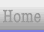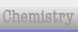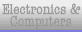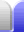# Comparing Surface Area and Volume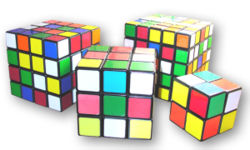### Chemical reations

The smaller an object is, the greater its surface area to volume ratio is. This is important for chemical reactions, since more surface area between the reactants will mean a faster reaction.

Let's compare the two simplest Rubik's Cubes in the picture above, and assume that each small block is 2 cm on a side. The 3 x 3 x 3 cube will then be 6 cm across, while the 2 x 2 cube will be 4 cm on a side.

3 x 3 x 3 cube:
surface area = 6 faces x 6 cm x 6cm = 216 cm2
volume = 6 cm x 6 cm x 6cm = 216 cm3
ratio of surface area:volume = 1:1

2 x 2 x 2 cube:
surface area = 6 faces x 4 cm x 4 cm = 96 cm2
volume = 4 cm x 4 cm x 4cm = 64 cm3
ratio of surface area:volume = 3:2

So in a reaction with particles similar to these Rubik's cubes, the smaller particles, 2/3 the size of the larger ones, would react 50% faster (all else being equal).

If we had one litre of particles, if it was just one solid cubic lump it would have a surface area of 600 cm2, but if it was ground up into cubic lumps one millimeter on a side we would have a surface area of 0.06 cm2 per lump, but with a million of these in a litre the total surface area would be 60,000 cm2, which would produce a much faster reaction.

Note that a faster reaction isn't always what we want. If a reaction occurs too quickly it can be very dangerous. In that case we would want to have larger particles, to slow the reaction down. We take advantage of this when we put a large log on a log fire before going to bed. The bigger the log, the slower it'll burn.

### Hot air balloons

Going the other way, the larger an object is, the lower its surface area to volume ratio is. This is important for hot air balloons, since less surface area per volume means less comparative weight of the balloon for the hot air it contains, which means more lifting power for the weight.

If we have a cubical balloon 1 metre across we'll have 1 m3 of hot air for (roughly) 6 m2 of balloon (1:6 ratio). For a cubic balloon 2 m on a side, we'll have 8 m3 of hot air for 24 m2 of balloon surface area – twice as much lifting power (1:3 ratio). A cube-shaped balloon just 10 cm on a side will contain 1 L (0.001 m3) of hot air and have a surface area of 0.06 m2much less lifting power (1:60 ratio).

When Mythbusters made a helium balloon out of lead foil (thereby disproving the idea that a lead balloon will invariably sink) they had to make it reasonably large – three metres across – because their first attempt didn't have enough lift (volume of helium) for the amount of lead weight (surface area).Home Astronomy Chemistry Electronics & Computers Mathematics Physics Field Trips Turn on javascript for email link